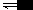# 化学自习室移动版

### 溶液中的离子浓度大小比较各种题型总结分析

1.电离理论：

⑴弱电解质的电离是微弱的，电离消耗的电解质及产生的微粒都是少量的，同时注意考虑水的电离的存在；

NH3・H2O溶液中微粒浓度大小关系:c(NH3・H2O)＞c(OH)＞c(NH4)＞c(H)。

⑵多元弱酸的电离是分步的，主要以第一步电离为主；

H2CO3溶液中微粒浓度大小关系:c(H2CO3)＞c(H)＞c(H CO3)＞c(CO32)c(OH)。

2.水解理论：

⑴弱酸的阴离子和弱碱的阳离子因水解而损耗；如NaHCO3溶液中有：c(Na)＞c(HCO3)。

⑵弱酸的阴离子和弱碱的阳离子的水解是微量的(双水解除外)，因此水解生成的弱电解质及产生H的(或OH)也是微量，但由于水的电离平衡和盐类水解平衡的存在，所以水解后的酸性溶液中c(H)(或碱性溶液中的c(OH))总是大于水解产生的弱电解质的浓度；

⑶一般来说“谁弱谁水解，谁强显谁性”，如水解呈酸性的溶液中c(H)＞c(OH)，水解呈碱性的溶液中c(OH)＞c(H)；

⑷多元弱酸的酸根离子的水解是分步进行的，主要以第一步水解为主。

Na2CO3溶液中微粒浓度关系:c(Na)＞c(CO32)＞c(OH)＞c(HCO3)＞c(H)。

1．电荷守恒：电解质溶液中所有阳离子所带有的正电荷数与所有的阴离子所带的负电荷数相等。

2．物料守恒：电解质溶液中由于电离或水解因素，离子会发生变化变成其它离子或分子等，但离子或分子中某种特定元素的原子的总数是不会改变的。

【注意】书写电荷守恒式必须①准确的判断溶液中离子的种类；②弄清离子浓度和电荷浓度的关系。

3．导出式－－质子守恒：

［规律总结］

1、必须有正确的思路：

2、掌握解此类题的三个思维基点：电离、水解和守恒

3、分清他们的主要地位和次要地位

【常见题型】

1.弱酸溶液：

【例1】在0.1mol/L 的H2S溶液中，下列关系错误的是()

A.c(H)=c(HS)＋c(S2-)＋c(OH)

B.c(H)＞c(HS)＞c(S2-)＞c(OH)

C.c(H)＞[c(HS)＋c(S2-)＋c(OH)]

D.c(H2S)＋c(HS)＋c(S2-)=0.1mol/L

2.弱碱溶液：

【例2】室温下，0.1mol/L 的氨水溶液中，下列关系式中不正确的是()

A. c(OH)＞c(H

B.c(NH3・H2O)＋c(NH4)=0.1mol/L

C.c(NH4)＞c(NH3・H2O)＞c(OH)＞c(H

D.c(OH)=c(NH4)＋c(H)

3.强酸弱碱盐溶液：

【例3】在氯化铵溶液中，下列关系正确的是()

A.c(Cl)＞c(NH4)＞c(H)＞c(OH

B.C(NH4)＞c(Cl)＞c(H)＞c(OH)

C.C(NH4)＝c(Cl)＞c(H)＝c(OH

D.c(Cl)＝c(NH4)＞c(H)＞c(OH)

4.强碱弱酸盐溶液：

【例4】在Na2S溶液中下列关系不正确的是()

A.c(Na) =2c(HS) ＋2c(S2-) ＋c(H2S)

B．c(Na) ＋c(H)=c(OH)＋c(HS)＋2c(S2-)

C．c(Na)＞c(S2-)＞c(OH)＞c(HS)

D．c(OH)=c(HS)＋c(H)＋c(H2S)

5.强碱弱酸的酸式盐溶液：

【例5】草酸是二元弱酸草酸氢钾溶液呈酸性在0.1mol/L KHC2O4溶液中，下列关系正确的是()

A．c(K)＋c(H)=c(HC2O4)＋c(OH)＋c(C2O42)

B．c(HC2O4)＋c(C2O42)=0.1mol/L

C．c(C2O42)＞c(H2C2O4)

D．c(K)=c(H2C2O4)＋c(HC2O4)＋c(C2O42)

1、两种物质混合不反应：

【例】：用物质的量都是0.1 mol 的CH3COOH和CH3COONa配制成1L 混合溶液，已知其中c(CH3COO)＞c(Na)，对该混合溶液的下列判断正确的是( )

A.c(H)＞c(OH

B.c(CH3COOH)＋c(CH3COO)＝0.2 mol/L

C.c(CH3COOH)＞c(CH3COO

D.c(CH3COO)＋c(OH)＝0.2 mol/L

2、两种物质恰好完全反应

【例】在10mL 0.1mol・L－1NaOH溶液中加入同体积、同浓度CH3COOH溶液，反应后溶液中各微粒的浓度关系错误的是()

A．c(Na)＞c(CH3COO)＞c(H)＞c(OH

B．c(Na)＞c(CH3COO)＞c(OH)＞c(H)

C．c(Na)＝c(CH3COO)＋c(CH3COOH)

D．c(Na)＋c(H)＝c(CH3COO)＋c(OH)

3、两种物质反应，其中一种有剩余：

(1)酸与碱反应型 关注所给物质的量是物质的量浓度还是pH

(解答此类题目时应抓住两溶液混合后剩余的弱酸或弱碱的电离程度和生成盐的水解程度的相对大小。)

【例1】：将0.2mol・L－1HCN溶液和0.1mol・L－1的NaOH溶液等体积混合后，溶液显碱性，下列关系式中正确的是 ()

A. c(HCN)＜c(CN)

B. c(Na)＞c(CN)

C. c(HCN)－c(CN)＝c(OH

D. c(HCN)＋c(CN)＝0.1mol・L－1

【例2】设氨水的pH＝X，某盐酸的pH＝Y，已知X＋Y＝14，且X＞11。将上述两溶液分别取等体积充分混合后，所得溶液中各离子浓度由大到小的顺序是

A. c(Cl)＞c(NH4)＞c(H)＞c(OH

B. c(NH4)＞c(Cl)＞c(H)＞c(OH)

C. c(NH4)＞c(Cl)＞c(OH)＞c(H

D. c(Cl)＞c(NH4)＞c(OH)＞c(H)

【例3】等体积等浓度的MOH强碱溶液和HA弱酸溶液混合后，混合溶液中有关离子浓度的关系正确的是()

A.c(M)＞c(OH)＞c(A)＞c(H

B. c(M)＞c(A)＞c(H)＞c(OH)

C.c(M)＞c(A)＞c(OH)＞c(H

D. c(M)＋ c(H) =c(A)＋ c(OH)

【例4】将标准状况下的2.24L CO2通入150mL1mol/LNaOH溶液中，下列说法正确的是()

A．c(HCO3)略大于c(CO32

B．c(HCO3)等于c(CO32)

C．c(Na)等于c(CO32)与c(HCO3)之和

D．c(HCO3)略小于c(CO32)

【例5】.常温下，将甲酸和氢氧化钠溶液混合，所得溶液pH＝7，则此溶液中()

A．c(HCOO)＞c(Na

B．c(HCOO)＜c(Na)

C．c(HCOO)＝c(Na

D．无法确定c(HCOO)与c(Na)的关系

A．[A]≥[M

B．[A]≤[M]

C．若MA不水解，则[OH]＜[A

D．若MA水解，则[OH]＞[A]

(2)盐与碱(酸)反应型

(解答此类题目时应抓住两溶液混合后生成的弱酸或弱碱的电离程度和剩余盐的水解程度的相对大小。)

【例1】.将0.1mol・L－1醋酸钠溶液20mL 与0.1mol・L－1盐酸10mL 混合后，溶液显酸性，则溶液中有关粒子浓度关系正确的是()

A．c(CH3COO)＞c(Cl)＞c(H)＞c(CH3COOH)

B．c(CH3COO)＞c(Cl)＞c(CH3COOH)＞c(H)

C．c(CH3COO)＝c(Cl)＞c(H)＞c(CH3COOH)

D．c(Na)＋c(H)＝c(CH3COO)＋c(Cl)＋c(OH)

【例2】下列混合溶液中，各离子浓度的大小顺序正确的是()

A . 10mL0・1mol・L－1氨水与10mL0・1mol・L－1盐酸混合：

c(Cl)＞c(NH4)＞c(OH)＞c(H)

B．10mL0・1mol・L－1NH4Cl与5mL0・2mol・L－1NaOH溶液混合：

c(Na)=c(Cl)＞c(OH)＞C(H)

C．10mL0・1mol・L－1CH3COOH溶液与5mL0・2mol・L－1NaOH溶液混合：

c(Na)=c(CH3COO)＞c(OH)＞c(H)

D．10mL0・5mol・L－1CH3COONa溶液与6mL1mol・L－1盐酸混合：

c(Cl)＞c(Na)＞c(OH)＞c(H)

【例3】物质的量浓度相同的200mL Ba(OH)2溶液和150mL NaHCO3混合后，最终溶液中离子的物质的量浓度关系正确的是()

A c(OH)＞c(Ba2＋)＞c(Na)＞c(CO32

B. c(OH)＞c(Na)＞c(Ba2＋)＞c(H)

C. c(OH)=c(Ba2＋)＋c(Na)＋c(H

D. c(H)＋c(Na)＋2c(Ba2＋)=c(OH)

n(OH)=8mol

n(Ba2＋)=4mol

n(Na)=3mol

n(HCO3)=3mol ；

4、不同物质同种离子浓度比较型：

【例题】物质的量浓度相同的下列溶液中，NH4浓度从大到小的顺序为  。

A．NH4Cl  B．NH4HSO C．CH3COONH4  D．NH4HCO3  E.( NH4)2SO4

5、其他类型

【例1】已知某无色透明的溶液中所含的离子仅有Na、CH3COO、H和OH四种离子，试回答下列问题：

⑴该溶液所含的四种离子的物质的量浓度大小之间的关系是否只有一种可能(填“是”或“否”)，其原因是：    。

⑵某学生将该溶液中的四种离子的物质的量浓度按从大到小的顺序排列了若干种可能，其中合理的是：

A. c(Na)＞c(CH3COO)＞c(OH)＞c(H

B. c(CH3COO)=c(Na)＞c(OH)=c(H)

C. c(H)＞c(OH)＞c(Na)＞c(CH3COO

D. c(Na)＞c(CH3COO)＞c(H)＞c(OH)

A.c(Cl)＞c(NH4)＞c(H)＞c(OH

B. c(Cl)＞c(H)＞c(NH4)＞c(OH)

C.c(Cl)＞c(NH4)＞c(OH)＞c(H

D. c(OH)＞c(H)＞c(Cl)＞c(NH4)

【例2】某二元酸(化学式用H2A表示)在水中的电离方程式是：H2A==H＋HA；HAH＋A2－回答下列问题：

⑴Na2A溶液显         (填“酸性”，“中性”，或“碱性”)。

⑵在0.1mol・L－1的Na2A溶液中，下列微粒浓度关系式正确的是：

A. c(A2－) ＋c( HA)＋c( H2A) =0.1mol・L－1

B. c(OH)=c(H) ＋c( HA)

C. c(Na)＋ c(H) =c(OH)＋ c( HA)＋2c(A2－

D. c(Na)= 2c(A2－) ＋2c( HA)

⑶已知0.1mol・L－1NaHA溶液的pH=2，则0.1mol・L－1H2A溶液中氢离子的物质的量浓度可能是          0.11 mol・L－1(填“＜”，“＞”，或“=”)，理由是：

⑷0.1mol・L－1NaHA溶液中各种离子浓度由大到小的顺序是：

【例3】今有一混合物的水溶液，只可能含有以下离子中的若干种：KNH4、Cl、Mg2＋、Fe3＋、CO32、SO42，现每次取100.00mL 进行实验。

①第一份加入AgNO3溶液有沉淀产生。②第二份加足量NaOH后加热，收集到气体0.896L(标准状态下)。③第三份加足量BaCl2后，得干燥沉淀6.27g，沉淀经足量盐酸洗涤干燥后，剩2.33g。

⑴判断K、Cl是否存在？K                 ；Cl－                (填下列序号)。

A．一定存在    B．可能存在    C．一定不存在

⑵肯定存在的离子的原溶液中的物质的量浓度分别为：

(责任编辑：化学自习室)• 全部评论（0
还没有评论，快来抢沙发吧！
相关文章
作者其他文章
最近关注
热点内容
公益广告